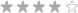Currency - All prices are in AUD

Currency - All prices are in AUDLoading... Please wait...

Consumables

• R870.00 (inc VAT) R756.52 (ex VAT)•• R185.00 (inc VAT) R160.87 (ex VAT)• R300.00 (inc VAT) R260.87 (ex VAT)• R440.00 (inc VAT) R382.61 (ex VAT)• R400.00 (inc VAT) R347.83 (ex VAT)• R225.00 (inc VAT) R195.65 (ex VAT)• R84.00 (inc VAT) R73.04 (ex VAT)• R45.95 (inc VAT) R39.96 (ex VAT)• R70.88 (inc VAT) R61.63 (ex VAT)• R216.25 (inc VAT) R188.04 (ex VAT)• R220.00 (inc VAT) R191.30 (ex VAT)• R70.88 (inc VAT) R61.63 (ex VAT)• R1,250.00 (inc VAT) R1,086.96 (ex VAT)•• R220.00 (inc VAT) R191.30 (ex VAT)• R678.00 (inc VAT) R589.57 (ex VAT)• R370.00 (inc VAT) R321.74 (ex VAT)• R172.00 (inc VAT) R149.57 (ex VAT)• R330.00 (inc VAT) R286.96 (ex VAT)• R82.00 (inc VAT) R71.30 (ex VAT)• R2,064.00 (inc VAT) R1,794.78 (ex VAT)• R185.00 (inc VAT) R160.87 (ex VAT)